# PSAT Math : How to find the missing number in a set

## Example Questions

### Example Question #1 : Sets

Which number completes the following series: 1, 2, 4, 8, 16, 32, 64, _?

128

64

16

Not enough information

15

128

Explanation:

All of the numbers in this series are 2n-1. The number that we are looking for is the eighth number. So 28–1 = 27 = 128.

### Example Question #2 : Sets

Alhough Danielle’s favorite flowers are tulips, she wants at least one each of three different kinds of flowers in her bouquet. Roses are twice as expensive as lilies and lilies are 25% of the price of tulips. If a rose costs $20 and Danielle only has$130, how many tulips can she buy?

4

1

2

3

5

2

Explanation:

She can only buy 2 tulips at \$80, because if she bought 3 she wouldn’t have enough to afford the other 2 kinds of flowers.  She has to spend at least 30 dollars (20 + 10) on 1 rose and 1 lily.

### Example Question #3 : Sets

Which of the following is not a rational number?

0.111...

1.75

√2

5

.001

√2

Explanation:

A rational number is a number that can be written in the form of a/b, where a and b are integers, aka a real number that can be written as a simple fraction or ratio. 4 of the 5 answer choices can be written as fractions and are thus rational.

5 = 5/1, 1.75 = 7/4, .001 = 1/1000, 0.111... = 1/9

√2 cannot be written as a fraction because it is irrational. The two most famous irrational numbers are √2 and pi.

### Example Question #4 : Sets

Which set represents all the single-digits integers (0-9) that are either prime, a perfect square, or found in the number 68?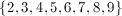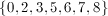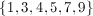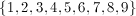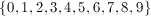Explanation:

The prime digits are 2, 3, 5, and 7.

The perfect square digits are 0, 1, 4, and 9.

The only digits not represent in these two groups are 6 and 8, which are, coincidentally, found in the number 68.

### Example Question #5 : Sets

If four different integers are selected, one from each of the following sets, what is the greater sum that these four integers could have?

W = {4, 6, 9, 10}

X = {4, 5, 8, 10}

Y = {3, 6, 7, 11}

Z = {5, 8, 10, 11}

42

41

38

40

39

38

Explanation:

By observing each of these sets, we can easily determine the largest number in each; however, the problem is asking for us to find the greatest possible sum if we select four different integers, one from each set. The largest number is 11. We will either select this number from set Y or set Z.

We will select set

Y for the 11

because the next larger number in set

Z is 10, which is greater than the next largest number is set Y, 7.

We can use set Z for 10.

Because the 10 has already been selected, choosing the integer from W and X should be easy because 9 is the next largest integer from set W

and

8 is the next largest integer from set X.

11 + 10 + 9 + 8 = 38

### Example Question #6 : Sets

A team has a win-loss ratio of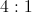. If the team wins six games in a row, the win-loss ratio will now be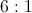. How many losses did the team have initially?Explanation:

If a team's ratio of wins to losses increases fromtoby winning six games, they must have 12 wins initially and 3 losses.

### Example Question #7 : Sets

Find the missing number in the following set: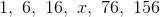Explanation:

The rule is as follows: add 2 to the previous number then multiply times 2.

The answer is (16 + 2) * 2 = 36.

### Example Question #1 : How To Find The Missing Number In A Set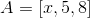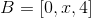If the average of Setis, what is the average of Set?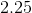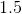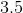Explanation:

We know the average of Set A is 5. Therefore: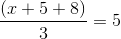Now let's solve for: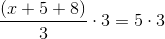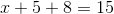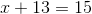We can now plug this value ofinto Set B and solve for the average:

Set B = {0, 2, 4}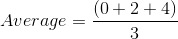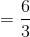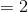The average of Set B is 2.